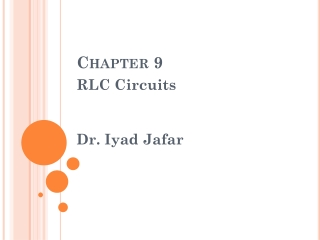Download PresentationChapter 9

# Chapter 9 - PowerPoint PPT PresentationDownload Presentation## Chapter 9

- - - - - - - - - - - - - - - - - - - - - - - - - - - E N D - - - - - - - - - - - - - - - - - - - - - - - - - - -
##### Presentation Transcript

1. Chapter 9 RLC Circuits Dr. Iyad Jafar

2. RLC Circuits • Analyze circuits containing R, L and C together • Series RLC • Parallel RLC • Similar to RL and RC circuits, RLC circuits has two parts • Source free or natural response • Forced / steady state response • Forced Response  a step input causes a step output. • Natural Response  Different and more difficult than RL, RC.

3. Second-order Differential equation Source-free RLC Circuits We study the natural response by studying source-free RLC circuits. Parallel Source-free RLC Circuit

4. This second-order differential equation can be solved by assuming solutions The solution should be in form of If the solution is good, then substitute it into the equation will be true. which means s=??

5. Use quadratic formula, we got Both and are solution to the equation Therefore, the complete solution is

6. From Define resonant frequency Damping factor Therefore, in which we divide into 3 cases according to the term inside the bracket

7. Solution to Second-order Differential Equations • α > ω0 (inside square root is a positive value) Overdamped case • α = ω0 (inside square root is zero) Critical damped case • α < ω0 (inside square root is a negative value) Underdamped case

8. 1. Overdamped case , α > ω0 Example: find v(t) if the initial conditions are vc(0) = 0, iL(0) = -10A α > ω0 ,therefore, this is an overdamped case  s1 = -1, s2 = -6

9. Then, we will use initial conditions to find A1, A2 From vc(0) = 0 we substitute t=0 … (1) From KCL  At t =0+ … (2)

10. Solve the equations (1) and (2) A1 = 84 A2 = -84 and the solution is v(t) t

11. 2. Critical damped case , α = ω0 Example: find v(t) if the initial conditions are vc(0) = 0, iL(0) = -10A α = ω0 , this is an critical damped case  s1 = s2 = -2.45 The complete solution of this case is in form of

12. Then, we will use initial conditions to find A1, A2 From vc(0) = 0 we substitute t=0 … (1) Therefore A2 =0 and the solution is reduced to Find A1 from KCL at t=0 … (2)

13. 3. Underdamped case , α < ω0 from The term inside the bracket will be negative and s will be a complex number define Then and

14. Use Euler’s Identity

15. 3. Underdamped case , α < ω0 Example: find v(t) if the initial conditions are vc(0) = 0, iL(0) = -10A α < ω0 ,therefore, this is an underdamped case and v(t) is in form where

16. Then, we will use initial conditions to find B1, B2 From vc(0+) = vc(0-) = 0 we substitute t=0 Therefore B1 =0 and the solution is reduced to Find B2 from KCL at t=0

17. Series RLC Overdamped Critical damped Underdamped Solution

18. Forced RLC Circuits Similar to RL and RC circuits, the total response is the sum of the transient/natural response and the forced/steady state response X(t) = Xn(t) + Xf(t) Where Xn is one of the three cases Overdamped Critical damped Underdamped And Xf(t) is the value as t ∞ and the constants are found from the initial conditions

19. Example Find vc(t) This is overdamped case, so the solution is in form

20. Consider the circuit we found that the initial conditions will be • vC(0) = 150 V • iL(0+) = iL(0-) = 5 A • And the steady state values • vC(∞) = 150 V • iL(∞) = 9 A Using vC(0) = 150 V , we got Using vC(∞) = 150 V , we got Therefore, 1. Vf = 150 2. A1+A2 = 0 ------------(1)

21. Use the initial condition iL(0) = 5 A, we have to change vc(t) to iL(t) From Next, use KCL on the circle below or

22. From Substitute iL(0) = 5A ------------------(2) From (1), (2) A1 = 13.5, A2 = -13.5 Therefore,